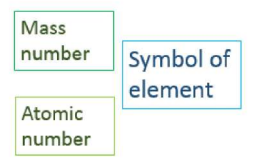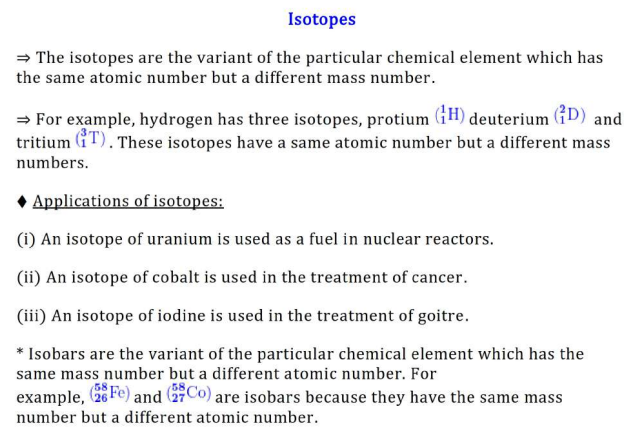# Structure of the Atom Class 9 Notes Science Chapter 4

By -

Structure of the Atom Class 9 Notes - We have provided a synopsis as well as revision notes for Class 9 Science Chapter 4. This set of CBSE notes includes CBSE Key Notes, CBSE Revision Notes, Short Key Notes, photos, and diagrams for the entire Chapter 4 titled Structure of the Atom taught in class 9. If you are a class 9 student utilising the NCERT Textbook to study Science, you will come across Chapter 4 Structure of the Atom. You must be looking for notes to memorise after you have studied the lecture. Complete Chapter 4 Structure of the Atom class 9 notes can be found here.

The free download of CBSE Class 9 Science Notes Chapter 4 Structure of the Atom Pdf is part of the Class 9 Science Notes for Quick Revision. NCERT Class 9 Science Notes Chapter 4 Structure of the Atom are provided here.

## CBSE Class 9 Science Notes Chapter 4 Structure of the Atom

Charged Particles in Matter

⇒ Atom is the building block of matter.

⇒ The matter is anything that has mass and takes up space.

⇒ Atoms are the smallest units of any matter. It cannot be seen through the naked eye.

⇒ These atoms are again subdivided into electrons, protons, and neutrons.

⇒ Protons were discovered by Ernest Rutherford, in his famous gold foil experiment. Electrons were discovered by J.J. Thomson, in his cathode ray tube experiment. Neutrons were discovered by James Chadwick.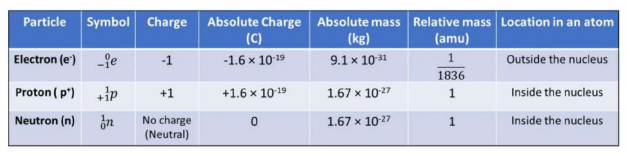The Structure of an Atom

⇒ An atom is the smallest particle on the earth of which matter is made up. But later on, Many atomic theories explain the existence of sub-atomic particles. These sub-atomic particles are electrons, protons, and neutrons.

Thomson's Model of an Atom:

J.J Thomson gave the first model for the structure of an atom. According to his model, the atoms consist of a positively charged sphere in which the electrons are embedded like the Christmas pudding. The atom is electrically neutral because the number of negative charges is equal in magnitude to the number of positive charges.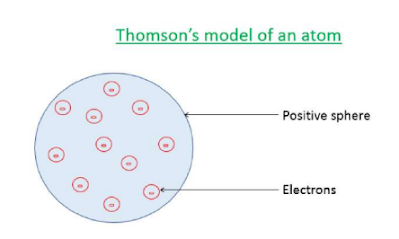Drawbacks of Thomson's model of an atom:

It doesn't explain the stability of an atom. It does not explain the nucleus of an atom.

Rutherford's Model of an Atom:

Rutherford performed the Gold foil experiment in which the fast-moving alpha particles were made to fall on the gold foil. Some of the alpha particles deflect at higher angles while some deflect at smaller angles and some went undeflected.

Rutherford's Gold Experiment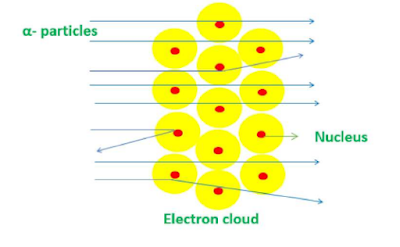According to Rutherford's model, the atom contains a nucleus at the centre which is heavy and positively charged. The size of the nucleus is very small compared to the size of the atom. The electron is a negatively charged particle that revolves around the nucleus. So, Rutherford discovered the nucleus.

→ Drawbacks of Rutherford's model of an atom:

The major drawback is the instability of the orbital revolution of an electron. If the electron moves around the nucleus at high speed, it will give up its energy and fall into the nucleus.

Bohr's Model of an Atom:

According to this model, the electrons revolve around the nucleus in discrete orbits called energy shells or energy levels. Each energy level is associated with a definite amount of energy. The change in energy takes place when the atom jumps from one energy level to another level. The energy level present in an atomic structure is shown below: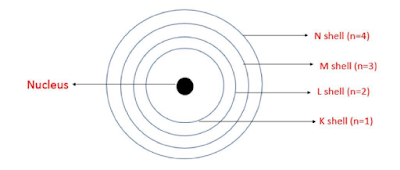Neutrons:

James Chadwick discovered the third subatomic particle, called the neutron. The neutron has no charge and it is present in the nucleus along with protons. The mass of the atom is given by the sum of neutrons and protons present in the nucleus.

How are Electrons Distributed in Different Orbits (Shells)?

Bohr and Bury suggested some rules for writing the number of electrons in different energy levels or shells. These rules are as follows: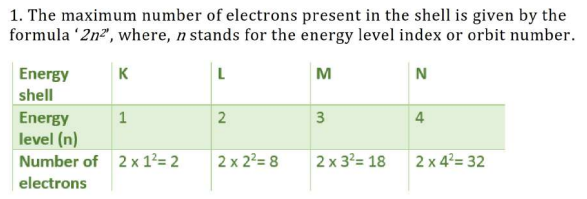2. The outermost orbit can accommodate a maximum of 8 electrons.

3. The orbits or the shells should be filled stepwise (first K shell will fill, then the L shell, and so on).

Valency

⇒ The octet rule refers to the tendency of atoms to prefer to have eight electrons in the valence shell.

⇒ The atoms which have fewer electrons than eight electrons react with another atom to achieve a stable octet.

The electrons present in the outermost shell of an atom are known as the valence electrons.

⇒ The combining capacity of the atoms or their tendency to react and form molecules with atoms of the same or different elements is known as the valency of the atom.

⇒ Valency is determined by subtracting valence electrons from the octet.

⇒ The valency of the atom is the same as that of the number of valence electrons in that atom for the atoms which have valence electrons less than or equal to 4. For example, beryllium contains 2 electrons in K-shell and 2 electrons in the L shell. So, its valency will be 2. The atomic structure of the beryllium is shown below: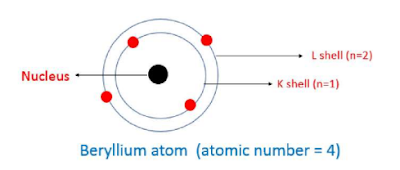⇒ The combining capacity or valency of an atom is zero which Atoms have completely filled the outermost shell. For example, neon is an inert gas that has a completely filled shell. So, its valency will be zero.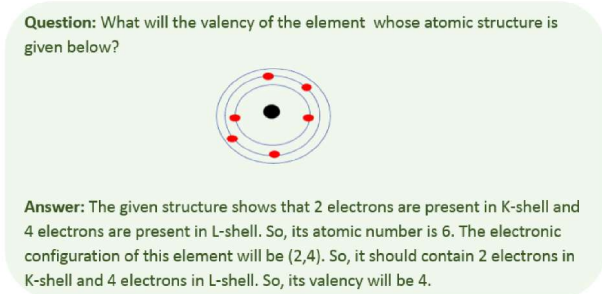⇒ The atomic number of the element is equal to the number of protons present in the nucleus of the atom.

⇒ The number of protons is equal to the number of electrons present in the atom.

Atomic number (Z)= Number of Protons = Number of Electrons

⇒ The mass number is defined as the total number of nucleons, which is the total number of protons and neutrons present in the nucleus of the atom.

Mass number (A)= Number of Protons + Number of Neutrons

⇒ The element is represented as shown below: